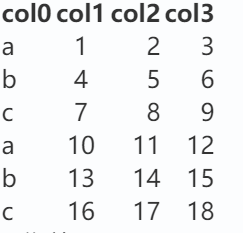``````  {
'a':[1, 2, 3]，[10, 11, 12]...
'b':[4, 5, 6]，[13, 14, 15]...
'c':[7, 8, 9]，[16, 17, 18]...
}
``````

``````def read_excel(bookname, sheetname):
# 打开Excel文件
wb = xlrd.open_workbook(bookname)
sheet = wb.sheet_by_name(sheetname)
dic = {}
for i in range(sheet.nrows - 1):
lis = []
for j in range(sheet.ncols):
lis.append(sheet.cell(i + 1, j).value)
dic[sheet.cell(i + 1, 0).value] = lis  #第一列作为key

print(dic)
return dic
``````

``````  {
'a':[10, 11, 12]
'b':[13, 14, 15]
'c':[16, 17, 18]
}
``````

1个回答